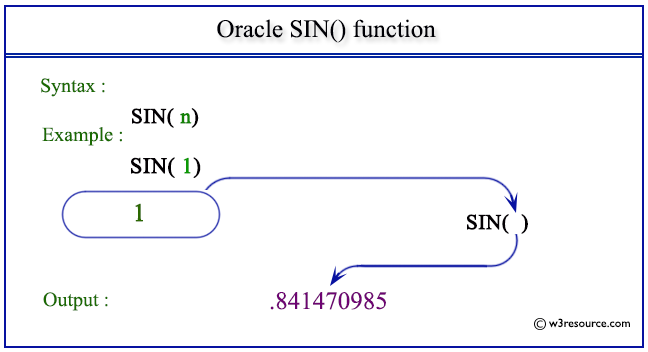# Oracle SIN() function

## Description

The SIN() function is used to get the sine of n (an angle expressed in radians).
The function takes any numeric or nonnumeric data type (can be implicitly converted to a numeric data type) as an argument.
If the argument is BINARY_FLOAT, then the function returns BINARY_DOUBLE. Otherwise, the function returns the same numeric data type as the argument.

Syntax:

`SIN(n)`

Parameters:

Name Description
n A number whose SIN value is to be retrieved.

Pictorial Presentation of SIN() functionExample:

``````SELECT SIN(1) FROM dual;
```
```

Here is the result.

```    SIN(1)
----------
.841470985
```

The above statement will return the sine of 1.

Previous: SIGN
Next: SINH

﻿